# Next greater element in an array

Given an array of integers(positive or negative), print the next greater element of all elements in the array. If there is no greater element then print null.
Next greater element of an array element array[i], is an integer array[j], such that
1. array[i] < array[j]
2. i < j
3. j - i is minimum
i.e. array[j] is the first element on the right of array[i] which is greater than array[i].
For example:
Input array:  98, 23, 54, 12, 20, 7, 27
Output:
Next greater element for 23     = 54
Next greater element for 12     = 20
Next greater element for 7     = 27
Next greater element for 20     = 27
Next greater element for 27     = null
Next greater element for 54     = null
Next greater element for 98     = null

## Algorithm/Insights

This problem can be solved by using a stack.
We traverse the array once.
1. If the stack is empty or the current element is smaller than top element of the stack, then push the current element on the stack.
2. If the current element is greater than top element of the stack, then this is the next greater element of the top element. Keep poping elements from the stack till a larger element than the current element is found on the stack or till the stack becomes empty. Push the current element on the stack.
3. Repeat steps 1 and 2 till the end of array is reached.
4. Finally pop remaining elements from the stack and print null for them.
Please note that at any instance, the stack will always be in sorted order having least element at the top and largest element at the bottom.

The algorithm is explained with the help of an example below: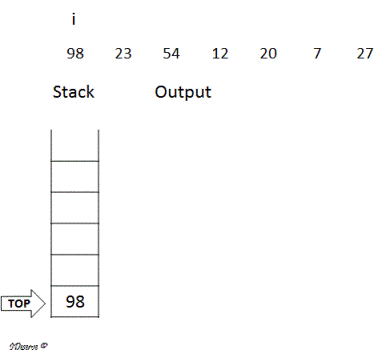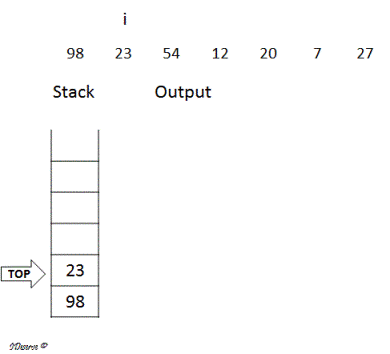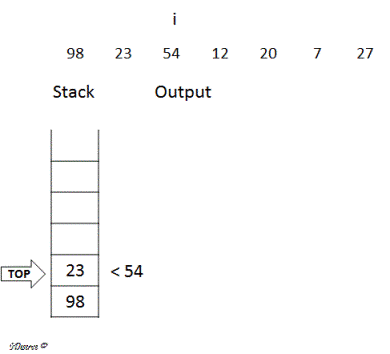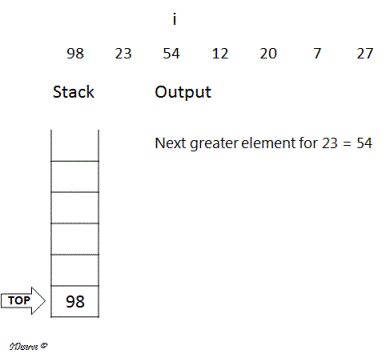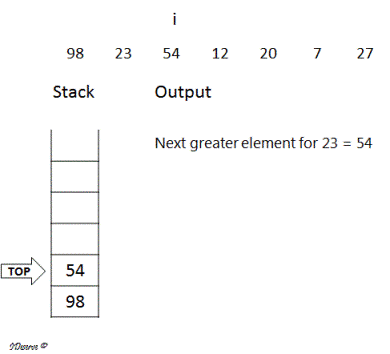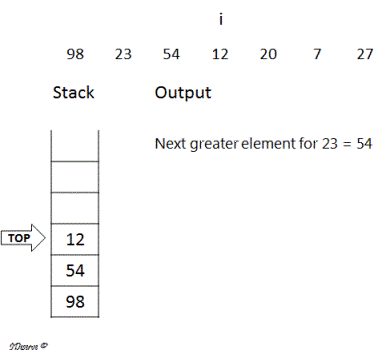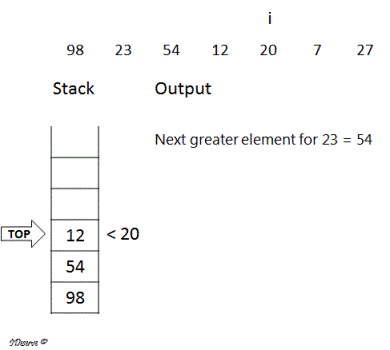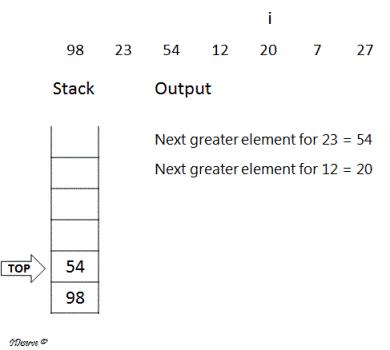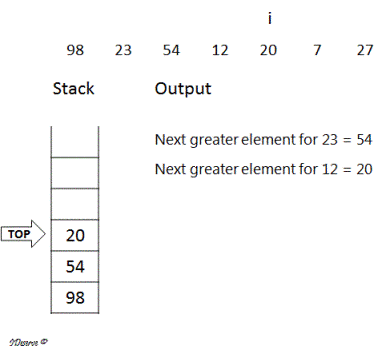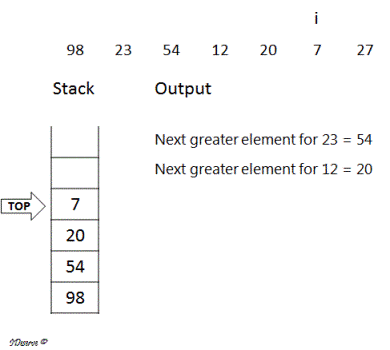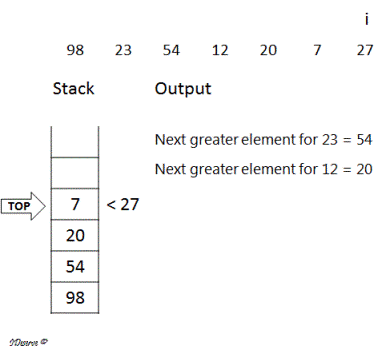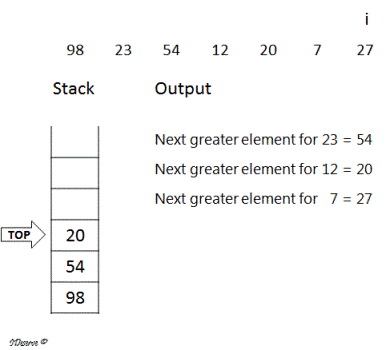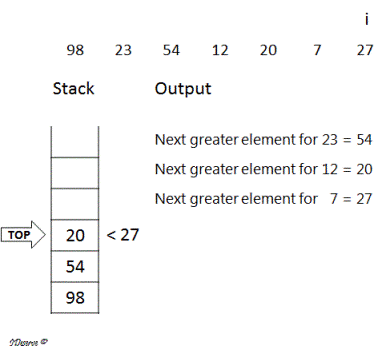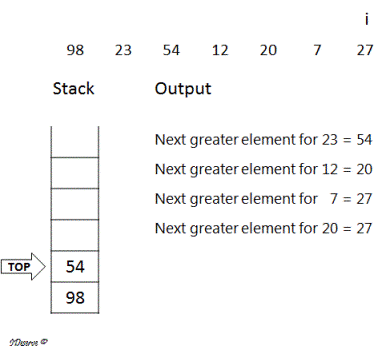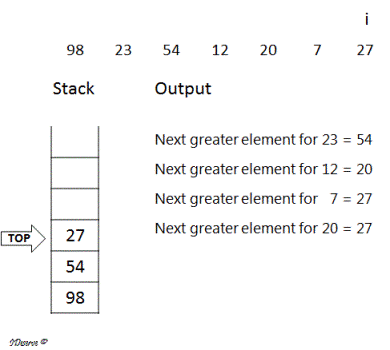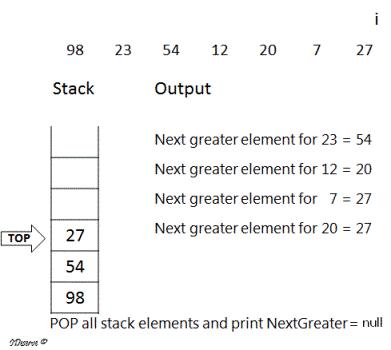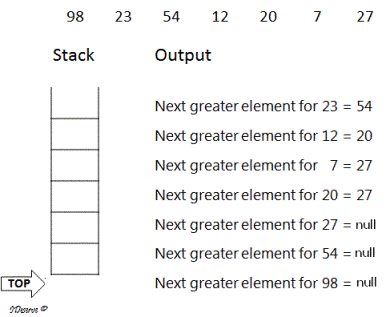## Code Snippet

```
package com.ideserve.questions.saurabh;

import java.util.Stack;

/**
* <b>IDeserve <br>
* Given an array of integers(positive or negative), print the next
* greater element of all elements in the array. If there is no greater
* element then print null.
*
* @author Saurabh
*/
public class NextGreaterElement {

public static void printNextGreaterElement(int[] input) {
Stack<Integer> stack = new Stack<Integer>();
int inputSize = input.length;
stack.push(input);
for (int i = 1; i < inputSize; i++) {
while (!stack.isEmpty() && stack.peek() < input[i]) {
System.out.println("Next greater element for "
+ stack.pop() + "\t = " + input[i]);
}
stack.push(input[i]);
}
while (!stack.isEmpty()) {
int top = (int) stack.pop();
System.out.println("Next greater element for " + top + "\t = " + null);
}
}

public static void main(String[] args) {
int[] input = { 98, 23, 54, 12, 20, 7, 27 };
printNextGreaterElement(input);
}
}
```

## Order of the Algorithm

Time Complexity is O(n) because each element is pushed 1 time and popped 1 time from the stack.
Space Complexity is O(n)